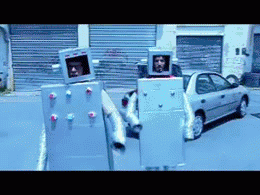# Advent of Code 2021: Day 3

## The Data

See the explanation for today’s challenge here. This time we need to work with binary numbers.We’re given a vector of numbers written in binary (e.g. 00100, 11110) and we need to use these to extract two values. It’s not as simple as just converting binary to decimal, we need to first generate new binary numbers that represent the most and least frequent value in each column. Let’s read the data in.

``````library(readr)

#Read in data where each binary number is a character string
day3_data <- readr::read_delim(file = "./data/Day3.txt", delim = "/t",
col_names = "binary", show_col_types = FALSE)

``````## # A tibble: 6 × 1
##   binary
##   <chr>
## 1 000010000011
## 2 001010000111
## 3 011010000010
## 4 000011111110
## 5 101101000101
## 6 000100010100``````

## The Challenges

### Challenge 1

To find the most and least common value in each column we first need to separate the character strings so that each binary bit is a separate column. We can do this using `separate()` in `tidyr` (and a bit of regex).

My first thought was just to use `separate()` where the separator is an empty string (““), but when we try this we end up with an erroneous empty column at the start.

``````library(tidyr)

binary_length <- nchar(day3_data\$binary)

day3_data %>%
separate(col = binary, into = as.character(1:binary_length), sep = "") %>%
``````## # A tibble: 6 × 12
##   `1`   `2`   `3`   `4`   `5`   `6`   `7`   `8`   `9`   `10`  `11`  `12`
##   <chr> <chr> <chr> <chr> <chr> <chr> <chr> <chr> <chr> <chr> <chr> <chr>
## 1 ""    0     0     0     0     1     0     0     0     0     0     1
## 2 ""    0     0     1     0     1     0     0     0     0     1     1
## 3 ""    0     1     1     0     1     0     0     0     0     0     1
## 4 ""    0     0     0     0     1     1     1     1     1     1     1
## 5 ""    1     0     1     1     0     1     0     0     0     1     0
## 6 ""    0     0     0     1     0     0     0     1     0     1     0``````

Instead, we can use regex to specify that we only want to separate by blank spaces that were preceded by a number. We can do this using the regex lookbehind operation `(?<=)`. In our example, by adding `(?<=[0-1])` we are specifying that a separator must have a number 0 or 1 preceding it.

``````separated_binary <- day3_data %>%
#Use convert = TRUE to automatically coerce to numeric
tidyr::separate(col = binary, into = as.character(1:binary_length), sep = "(?<=[0-1])", convert = TRUE)

``````## # A tibble: 6 × 12
##     `1`   `2`   `3`   `4`   `5`   `6`   `7`   `8`   `9`  `10`  `11`  `12`
##   <int> <int> <int> <int> <int> <int> <int> <int> <int> <int> <int> <int>
## 1     0     0     0     0     1     0     0     0     0     0     1     1
## 2     0     0     1     0     1     0     0     0     0     1     1     1
## 3     0     1     1     0     1     0     0     0     0     0     1     0
## 4     0     0     0     0     1     1     1     1     1     1     1     0
## 5     1     0     1     1     0     1     0     0     0     1     0     1
## 6     0     0     0     1     0     0     0     1     0     1     0     0``````

Now identifying the most or least common value is easy. If the sum of the column is greater than the number of rows, 1 is most common and visa-versa. Below we return TRUE if 1 is most common or FALSE when 0 is most common, which directly corresponds to 1 and 0 respectively when converted to an integer.

``````#Is 1 most common?
most_common <- separated_binary %>%
#Return sum of each col
#If it's greater than half nrow() then 1 is most common (and the inverse is true)
summarise(across(.cols = everything(), .fns = ~sum(.) > (n()/2)))

as.integer(most_common)``````
``##   1 0 1 1 1 1 0 0 1 1 1 0``

As a final step, we can use the `strtoi()` function to convert from binary to decimal. This function requires a single string input, so we need to convert our vector of most/least common numbers to a single character string.

``(most_common_binary <- paste(as.integer(most_common), collapse = ""))``
``##  "101111001110"``
``(least_common_binary <- paste(as.integer(!most_common), collapse = ""))``
``##  "010000110001"``
``````#Convert each number to decimal
(most_common_decimal    <- strtoi(most_common_binary, base = 2))``````
``##  3022``
``(least_common_decimal  <- strtoi(least_common_binary, base = 2))``
``##  1073``

Our answer is the product of these two numbers.

``most_common_decimal * least_common_decimal``
``##  3242606``

### Challenge 2

The second challenge is just a slightly more complex version of challenge 1, so I’m going to skip the explanation for now. If you’re interested, you can see the code on GitHub.

See previous solutions here:###### Post-doctoral researcher

Ecologist and data science, interested in using data science techniques for conservation outcomes.